# Build a Deep Audio De-Noiser Using TensorFlow 2.0Build a Deep Audio De-Noiser Using TensorFlow 2.0 .Speech denoising is a long-standing problem. Given a noisy input signal, the aim is to filter out such noise without degrading the signal of interest.

## Introduction

Speech denoising is a long-standing problem. Given a noisy input signal, the aim is to filter out such noise without degrading the signal of interest. You can imagine someone talking in a video conference while a piece of music is playing in the background. In this situation, a speech denoising system has the job of removing the background noise in order to improve the speech signal. Besides many other use cases, this application is especially important for video and audio conferences, where noise can significantly decrease speech intelligibility.

Classic solutions for speech denoising usually employ generative modeling. Here, statistical methods like Gaussian Mixtures estimate the noise of interest and then recover the noise-removed signal. However, recent development has shown that in situations where data is available, deep learning often outperforms these solutions.

In this article, we tackle the problem of speech denoising using Convolutional Neural Networks (CNNs). Given a noisy input signal, we aim to build a statistical model that can extract the clean signal (the source) and return it to the user. Here, we focus on source separation of regular speech signals from ten different types of noise often found in an urban street environment.

## Datasets

For the problem of speech denoising, we used two popular publicly available audio datasets.

As Mozilla puts it on the MCV website:

Common Voice is Mozilla’s initiative to help teach machines how real people speak.

The dataset contains as many as 2,454 recorded hours, spread in short MP3 files. The project is open source and anyone can collaborate on it. Here, we used the English portion of the data, which contains 30GB of 780 validated hours of speech. One very good characteristic of this dataset is the vast variability of speakers. It contains recordings of men and women from a large variety of ages and accents.

The UrbanSound8K dataset also contains small snippets (<=4s) of sounds. However, there are 8732 labeled examples of ten different commonly found urban sounds. The complete list includes:

• 0 = air_conditioner
• 1 = car_horn
• 2 = children_playing
• 3 = dog_bark
• 4 = drilling
• 5 = engine_idling
• 6 = gun_shot
• 7 = jackhammer
• 8 = siren
• 9 = street_music

As you might be imagining at this point, we’re going to use the urban sounds as noise signals to the speech examples. In other words, we first take a small speech signal — this can be someone speaking a random sentence from the MCV dataset.

Then, we add noise to it — such as a woman speaking and a dog barking on the background. Finally, we use this artificially noisy signal as the input to our deep learning model. The Neural Net, in turn, receives this noisy signal and tries to output a clean representation of it.

The image below displays a visual representation of a clean input signal from the MCV (top), a noise signal from the UrbanSound dataset (middle), and the resulting noisy input (bottom) — the input speech after adding the noise signal. Also, note that the noise power is set so that the signal-to-noise ratio (SNR) is zero dB (decibel). A ratio higher than 1:1 (greater than 0 dB) indicates more signal than noise.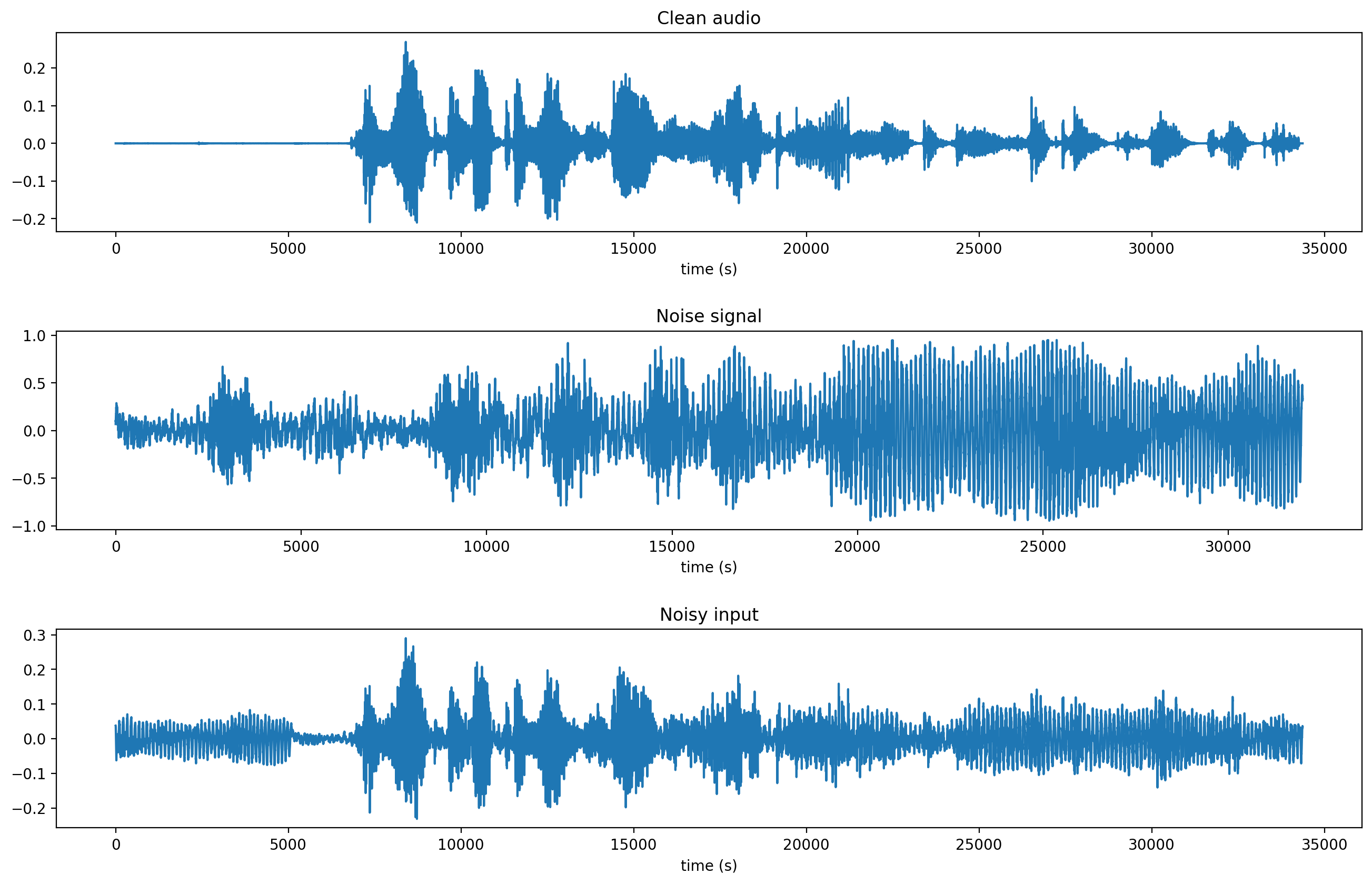## Data Preprocessing

Most of the benefits of current deep learning technology rest in the fact that hand-crafted features ceased to be an essential step to build a state-of-the-art model. Take feature extractors like SIFT and SURF as an example, which are often used in Computer Vision problems like panorama stitching. These methods extract features from local parts of an image to construct an internal representation of the image itself. However, to achieve the necessary goal of generalization, a vast amount of work is necessary to create features that were robust enough to apply to real-world scenarios. Put differently, these features needed to be invariant to common transformations that we often see day-to-day. Those might include variations in rotation, translation, scaling, and so on. One of the cool things about current deep learning is that most of these properties are learned either from the data and/or from special operations, like the convolution.

For audio processing, we also hope that the Neural Network will extract relevant features from the data. However, before feeding the raw signal to the network, we need to get it into the right format.

First, we downsampled the audio signals (from both datasets) to 8kHz and removed the silent frames from it. The goal is to reduce the amount of computation and dataset size.

It is important to note that audio data differs from images. Since one of our assumptions is to use CNNs (originally designed for Computer Vision) for audio denoising, it is important to be aware of such subtle differences. Audio data, in its raw form, is a one-dimensional time-series data. Images, on the other hand, are two-dimensional representations of an instant moment in time. For these reasons, audio signals are often transformed into (time/frequency) 2D representations.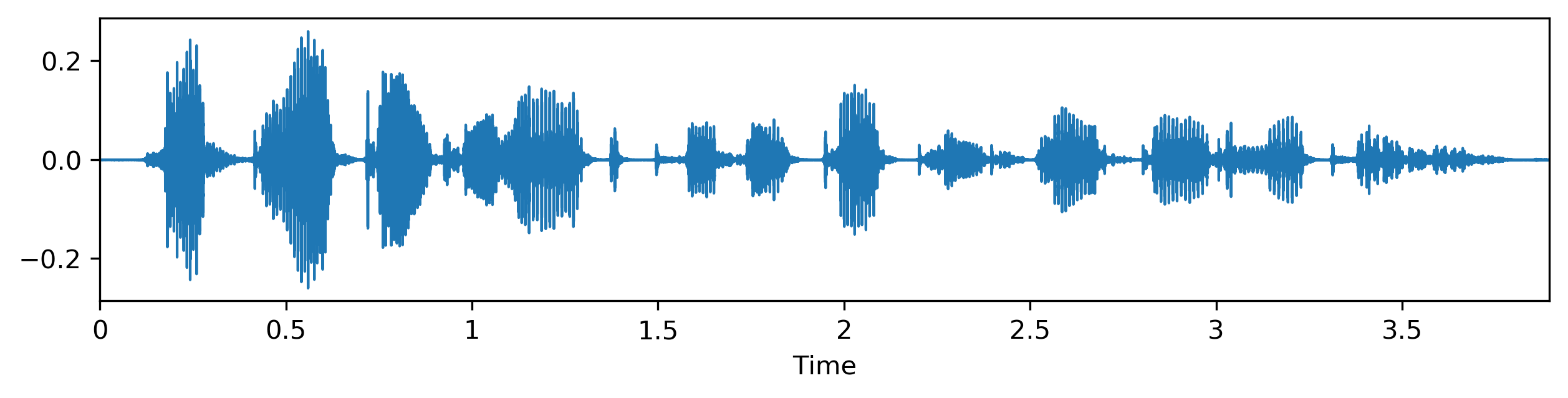The Mel-frequency Cepstral Coefficients (MFCCs) and the constant-Q spectrum are two popular representations often used on audio applications. For deep learning, classic MFCCs may be avoided because they remove a lot of information and do not preserve spatial relations. However, for source separation tasks, computation is often done in the time-frequency domain. Audio signals are, in their majority, non-stationary. In other words, the signal’s mean and variance are not constant over time. Thus, there is not much sense in computing a Fourier Transform over the entire audio signal. For this reason, we feed the DL system with spectral magnitude vectors computed using a 256-point Short Time Fourier Transform (STFT). You can see common representations of audio signals below.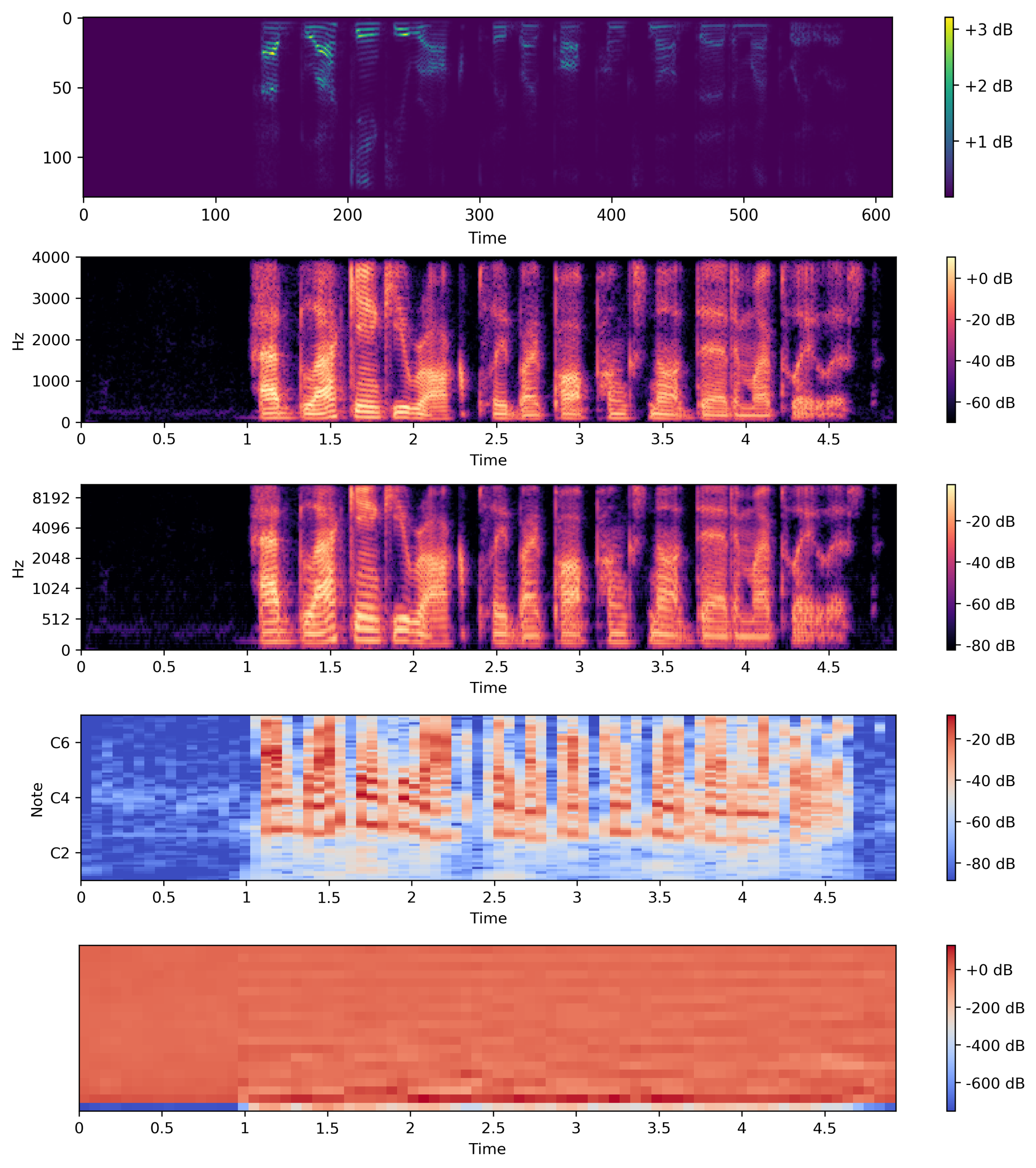To calculate the STFT of a signal, we need to define a window of length M and a hop size value R. The latter defines how the window moves over the signal. Then, we slide the window over the signal and calculate the discrete Fourier Transform (DFT) of the data within the window. Thus, the STFT is simply the application of the Fourier Transform over different portions of the data. Lastly, we extract the magnitude vectors from the 256-point STFT vectors and take the first 129-point by removing the symmetric half. All this process was done using the Python Librosa library. The image below, from MATLAB, illustrates the process.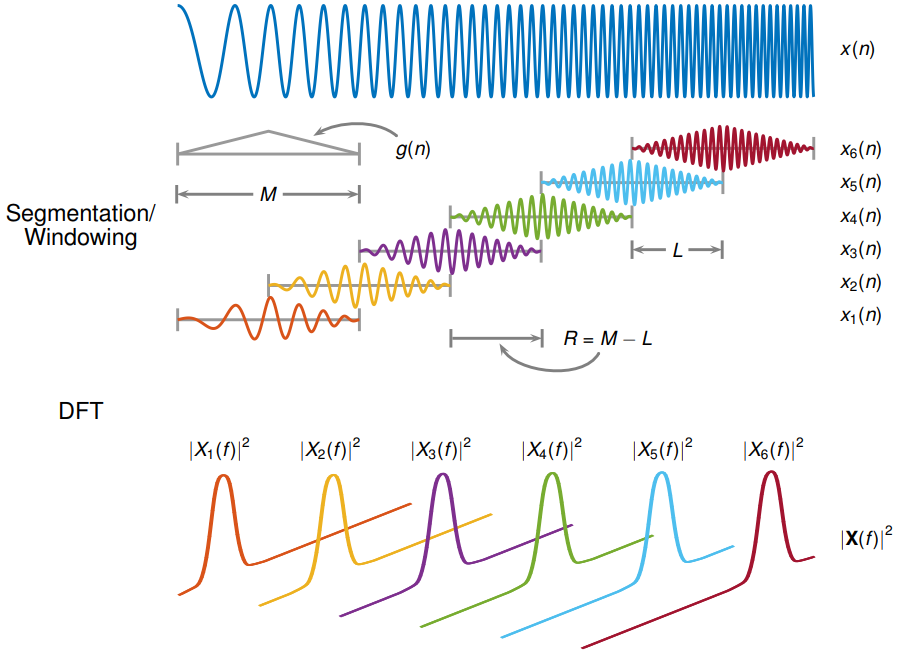Credits: MATLAB STFT docs

Here, we defined the STFT window as a periodic Hamming Window with length 256 and hop size of 64. This ensures a 75% overlap between the STFT vectors. In the end, we concatenate eight consecutive noisy STFT vectors and use them as inputs. Thus, an input vector has a shape of (129,8) and is composed of the current STFT noisy vector plus seven previous noisy STFT vectors. In other words, the model is an autoregressive system that predicts the current signal based on past observations. Therefore, the targets consist of a single STFT frequency representation of shape (129,1) from the clean audio. The image below depicts the feature vector creation.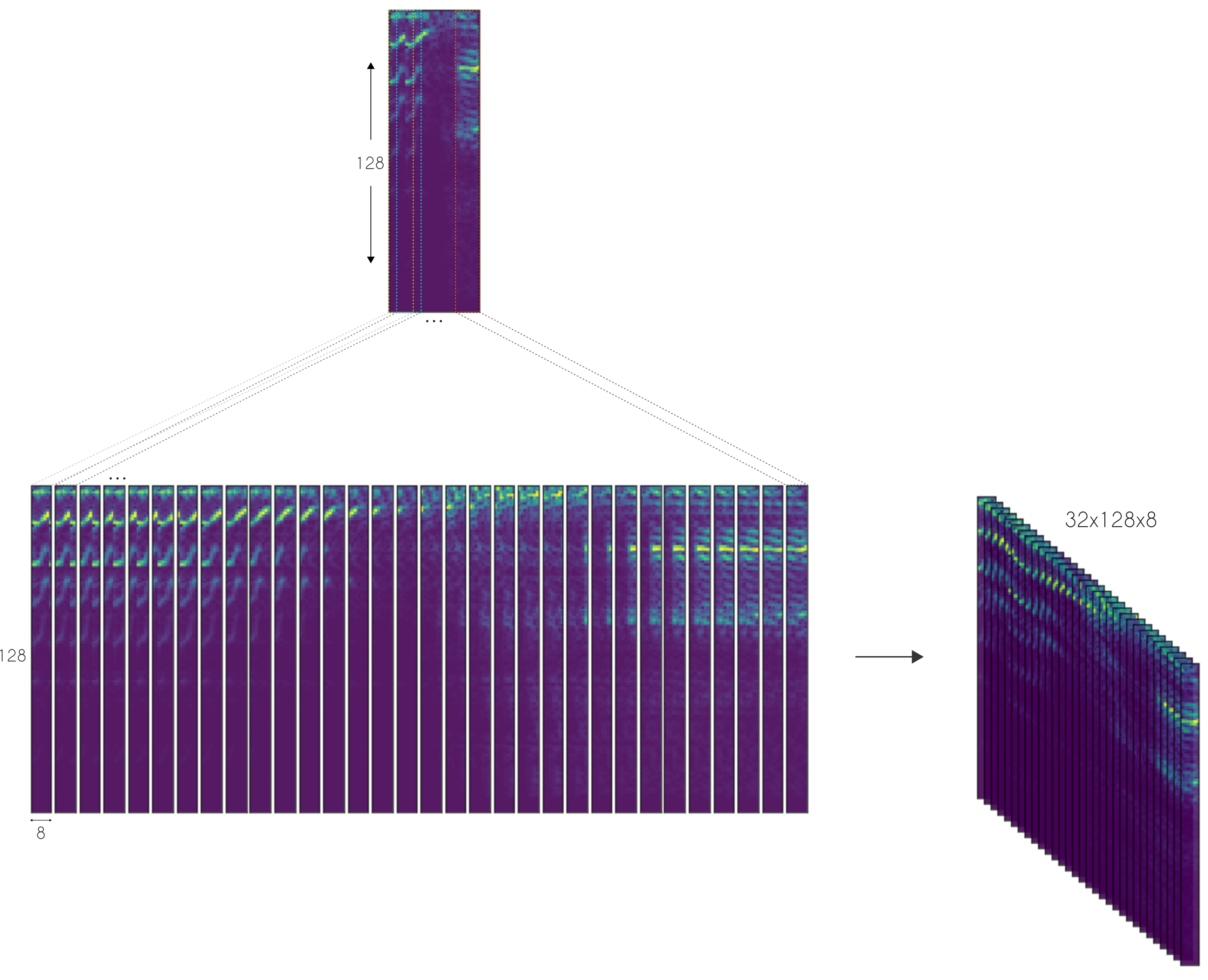## Deep Learning Architecture

Our Deep Convolutional Neural Network (DCNN) is largely based on the work done by A Fully Convolutional Neural Network for Speech Enhancement. Here, the authors propose the Cascaded Redundant Convolutional Encoder-Decoder Network (CR-CED).

The model is based on symmetric encoder-decoder architectures. Both components contain repeated blocks of Convolution, ReLU, and Batch Normalization. In total, the network contains 16 of such blocks — which adds up to 33K parameters.

Also, there are skip connections between some of the encoder and decoder blocks. Here the feature vectors from both components are combined through addition. Very much like ResNets, the skip connections speed up convergence and reduces the vanishing of gradients.

Another important characteristic of the CR-CED network is that convolution is only done in one dimension. More specifically, given an input spectrum of shape (129 x 8), convolution is only performed in the frequency axis (i.e the first one). This ensures that the frequency axis remains constant during forwarding propagation.

The combination of a small number of training parameters and model architecture, makes this model super lightweight, with fast execution, especially on mobile or edge devices.

Once the network produces an output estimate, we optimize (minimize) the mean squared difference (MSE) between the output and the target (clean audio) signals.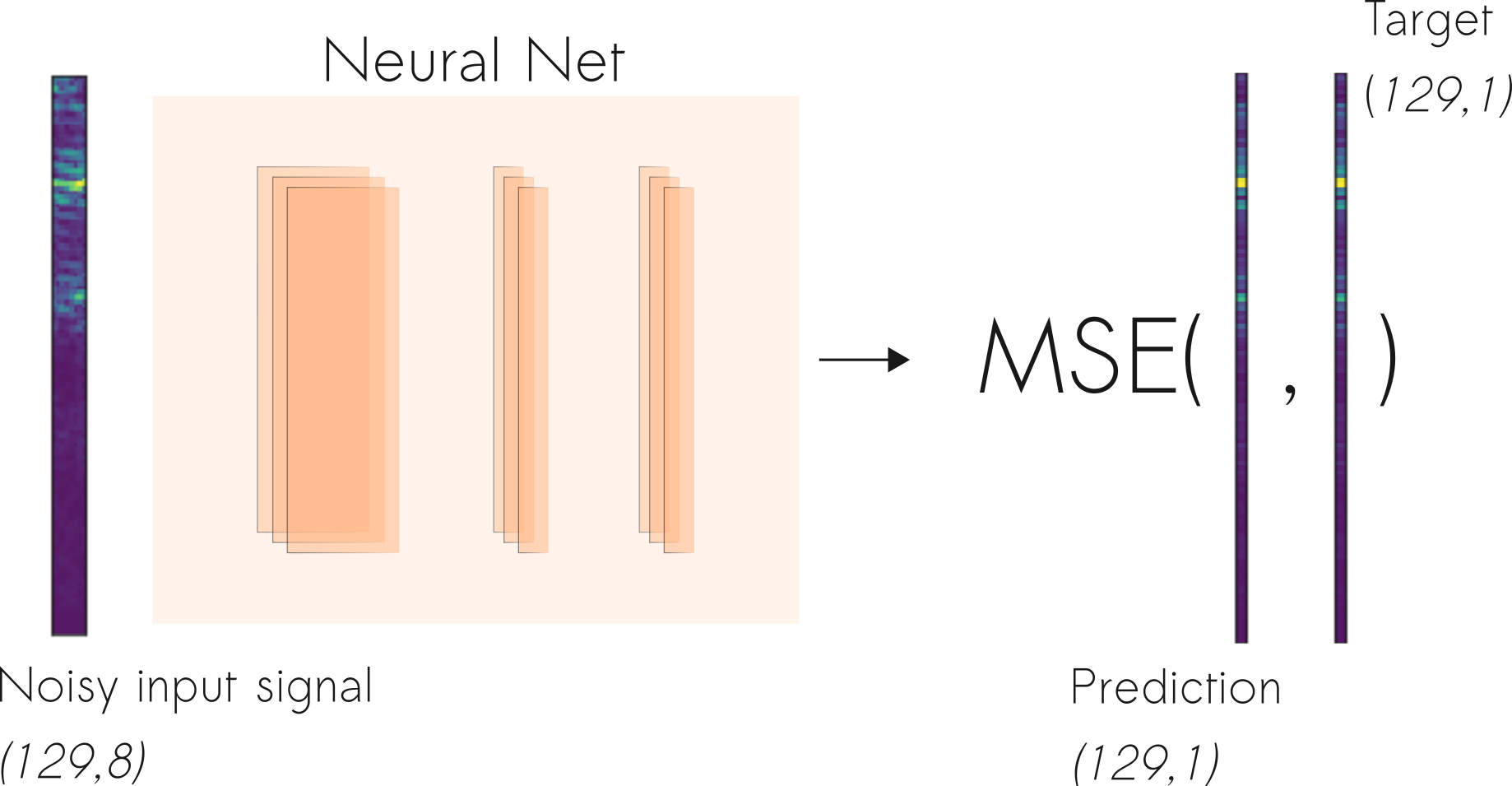## Results and Discussion

Let’s check some of the results achieved by the CNN denoiser.

To begin, listen to test examples from the MCV and UrbanSound datasets. They are the clean speech and noise signal, respectively. To recap, the clean signal is used as the target, while the noise audio is used as the source of the noise.

If you are having trouble listening to the samples, you can access the raw files here.

Now, take a look at the noisy signal passed as input to the model and the respective denoised result.

Below, you can compare the denoised CNN estimation (bottom) with the target (clean signal on the top) and noisy signal (used as input in the middle).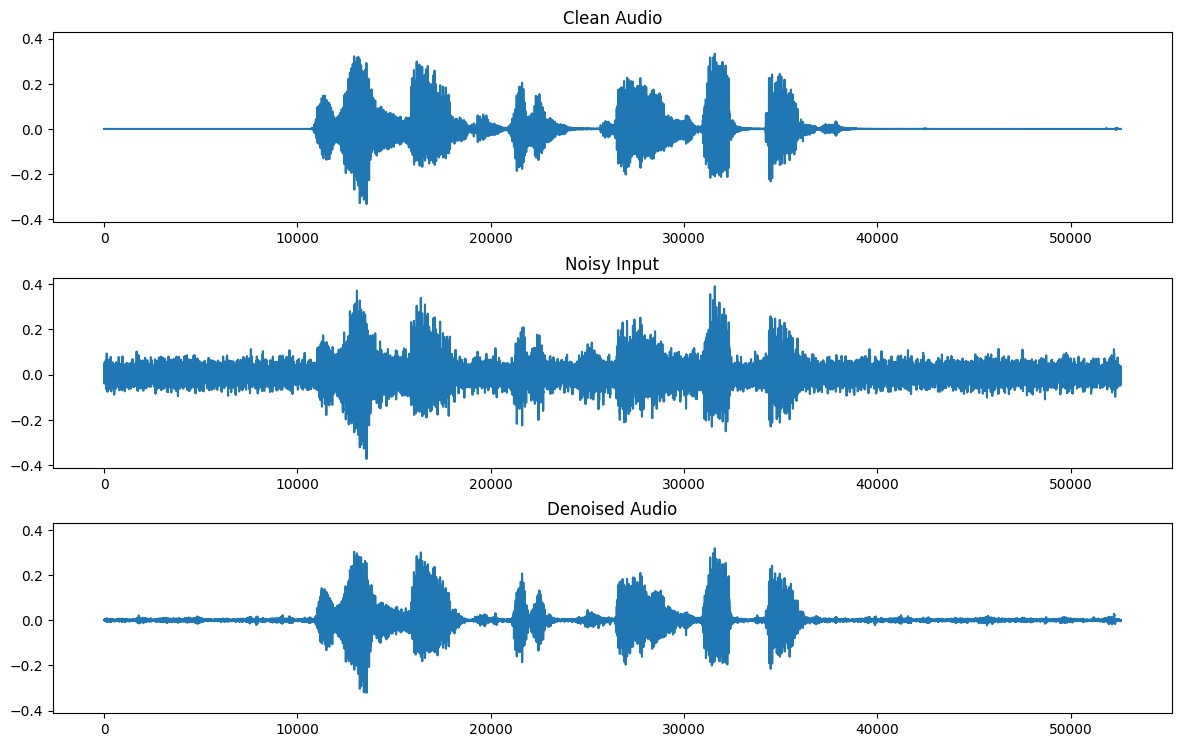As you can see, given the difficulty of the task, the results are somewhat acceptable, but not perfect. Indeed, in most of the examples, the model manages to smooth the noise but it doesn’t get rid of it completely. Take a look at a different example, this time with a dog barking in the background.

One of the reasons this prevents better estimates is the loss function. The Mean Squared Error (MSE) cost optimizes the average over the training examples. We can think of it as finding the mean model that smooths the input noisy audio to provide an estimate of the clean signal. Therefore, one of the solutions is to devise more specific loss functions to the task of source separation.

A particularly interesting possibility is to learn the loss function itself using GANs (Generative Adversarial Networks). Indeed, the problem of audio denoising can be framed as a signal-to-signal translation problem. Very much like image-to-image translation, first, a Generator network receives a noisy signal and outputs an estimate of the clean signal. Then, the Discriminator net receives the noisy input as well as the generator predictor or the real target signals. This way, the GAN will be able to learn the appropriate loss function to map input noisy signals to their respective clean counterparts. That is an interesting possibility that we look forward to implementing.

## Conclusion

Audio denoising is a long-standing problem. By following the approach described in this article, we reached acceptable results with relatively small effort. The benefit of a lightweight model makes it interesting for edge applications. As a next step, we hope to explore new loss functions and model training procedures.

You can get the full code here.

## What is TensorFlow? TensorFlow

An end-to-end open-source platform for Machine Learning. Before we start with TensorFlow, we will need to know what machine learning and deep learning technologies are.

## A TensorFlow Modeling Pipeline using TensorFlow Datasets and TensorBoard

This article investigates TensorFlow components for building a toolset to make modeling evaluation more efficient. Specifically, TensorFlow Datasets (TFDS) and TensorBoard (TB) can be quite helpful in this task.

## Keras vs. Tensorflow - Difference Between Tensorflow and Keras

Keras vs Tensorflow - Learn the differences between Keras and Tensorflow on basis of Ease to use, Fast development,Functionality,flexibility,Performance etc

## A TensorFlow Modeling using TensorFlow Datasets and TensorBoard

While completing a highly informative AICamp online class taught by Tyler Elliot Bettilyon (TEB) called Deep Learning for Developers, I …

## What is Tensorflow | Tensorflow for beginners (2020)

This Tensorflow tutorial beginners will help you understand what is Tensorflow in a very simplified manner for beginners. TensorFlow is one of the best libraries to implement Deep Learning. Deep learning is part of a broader family of machine learning methods based on artificial neural networks with representation learning. Learning can be supervised, semi-supervised or unsupervised.